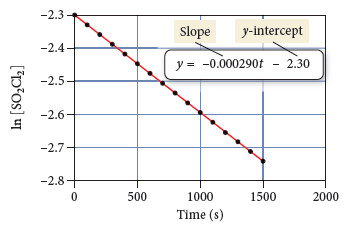# Problem: Consider the equation for the decomposition of SO2Cl2: SO2Cl2(g) → SO2(g) + Cl2(g)The graph of ln [SO2Cl2] versus time isUse the graph and the best fitting line to predict the concentration of SO2Cl2 at 1800 s.

###### FREE Expert Solution

Based on the graph:

y = ln [SO2Cl2]

x = time

The equation of the line is in the form of y = mx + b.

y = –0.000290t – 2.30

ln [SO2Cl2] = –0.000290t – 2.30

We can now predict the concentration of SO2Cl2 at 1800 s.

t = 1800 s###### Problem Details

Consider the equation for the decomposition of SO2Cl2SO2Cl2(g) → SO2(g) + Cl2(g)

The graph of ln [SO2Cl2] versus time isUse the graph and the best fitting line to predict the concentration of SO2Cl2 at 1800 s.